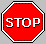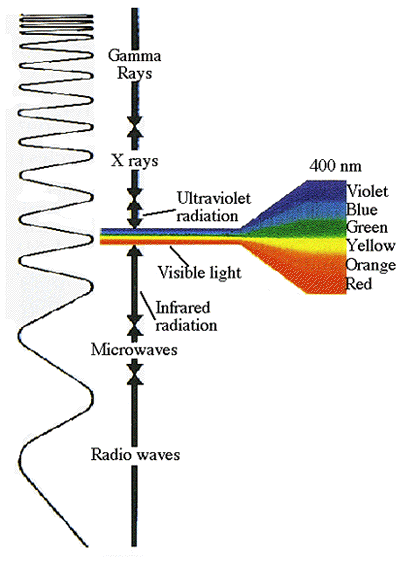# EXPERIMENT #3: STOP THAT GAMMA

## Introduction

This activity is very similar to the experiments #1 and #2 and may give better results.Gamma rays are high energy electromagnetic radiation emitted as a result of transition of a high energy level of the nucleus to a lower level. These emissions of high energy particles from the nucleus are also called photons. Gamma radiation has a higher penetration power than alpha and beta radiation.
Because of fluctuations of the meter, it is difficult to obtain an extremely accurate count of radiation with the scales set to x1. One must count the audible beats.
The source of gamma radiation for the experiment is Cobalt-60 (Co-60). In this experiment, the distances are 8 cm, 16 cm, and 24 cm from the source. 8 cm equal one data point, 16 cm equals two data points and so on.

## Objective

The purpose of this experiment is to find the range of gamma rays and determine if the inverse square law applies.

## Materials

• Geiger counter
• rail tracker
• Co-60 (gamma source)
• stop watch/beeper
• counting paper or hand counter
• graph paper

## Procedure

1. Place gamma radiation source into hole on wooden block of rail tracker.
2. Set digital Geiger counter to one minute intervals and turn power on. If you have an analog Geiger counter, it may be necessary to count the audible beats. Allow the instrument to warm up for a few minutes.
3. Record background activity.
4. Place the instrument on the slider, 8 cm from the source.
5. Record counts per minute (cpm) in Table 3.1 for each trial and calculate the average.
6. Move Geiger counter and slider to 16 cm from source. Repeat step 5.
7. Move Geiger counter to 24 cm and repeat step 5.
8. Calculate the uncertainty as the square root of the number of counts. Make sure you add the number of counts, take the square root and then divide by the total time. Record the calculation in column 8 of the Data Table. The plus or minus of this number will indicate the standard error of the experiment. This will be used to indicate the standard error of data in the experiment.

## Data

### Table 3.1: GAMMA RAYS-DISTANCE (cm)

Background __________ cpm     Co-60     Time=60 s

Distance (cm) Data Points r2 Trial 1 Trial 2 Trial 3 Average (cpm) Uncertainty (1/r2) average count of first data point
8 1 1
16 2 4
24 3 9

1. Graph the activity readings (cpm) vs. distance.
2. Graph the activity readings (cpm) vs. 1/r2.

## Questions

1. In what ways are light waves and gamma radiation related?
2. Where does the energy of the photons originate?
3. How are photons and X-rays alike? Different?
4. What happens to the intensity of gamma radiation between 8 cm and 24 cm?

## Going Further

1. What is the speed of gamma rays?
2. Make a graph combining the data of 1/r2 vs. cpm for experiments 1, 2, and 3.

This document was produced by the Nuclear Science Division of the Lawrence Berkeley National Laboratory.